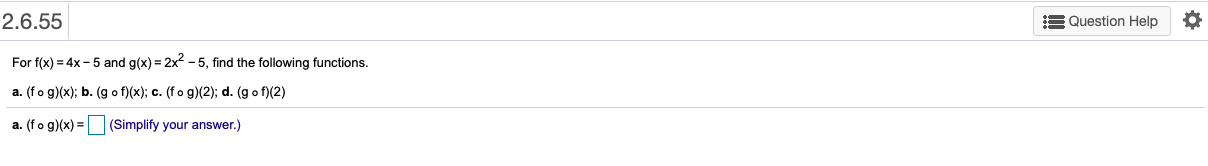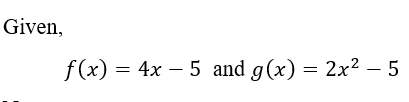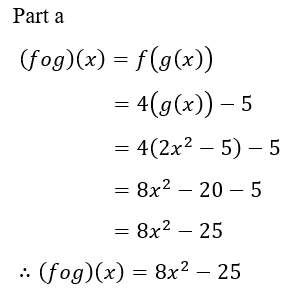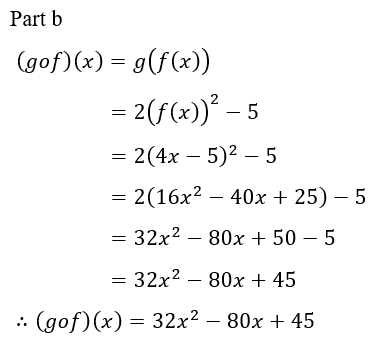# 2.6.55E Question HelpFor f(x) = 4x - 5 and g(x) = 2x - 5, find the following functions.a. (fo g)(x); b. (g o f)(x); c. (fo g)(2); d. (g o f)(2)a. (fo g)(x) =(Simplify your answer.)

Question
1 views

help with part

a

b

c

dhelp_outlineImage Transcriptionclose2.6.55 E Question Help For f(x) = 4x - 5 and g(x) = 2x - 5, find the following functions. a. (fo g)(x); b. (g o f)(x); c. (fo g)(2); d. (g o f)(2) a. (fo g)(x) = (Simplify your answer.) fullscreen
check_circle

star
star
star
star
star
1 Rating
Step 1Step 2Step 3...

### Want to see the full answer?

See Solution

#### Want to see this answer and more?

Solutions are written by subject experts who are available 24/7. Questions are typically answered within 1 hour.*

See Solution
*Response times may vary by subject and question.
Tagged in